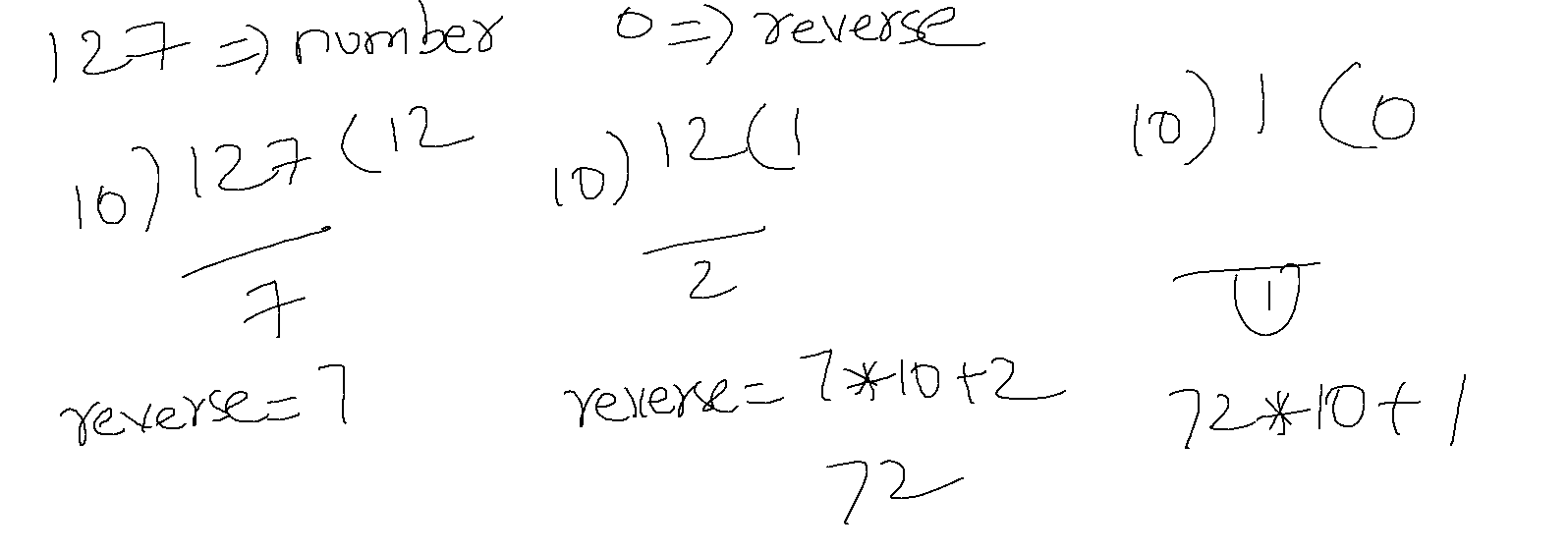## Instruct ELSA to find the number is pallendrome or not

• Logic:• Instructions:
``````1. Remember input as number and temp_number
2. reverse = 0
3. last_digit = number%10
4. reverse = last_digit + reverse * 10
5. number = number/10
6. go to step 3 if number != 0
7. say reverse
8 if reverse == temp_number say pallendrome
``````

## BASH

• To Remember
``````number=5
name='shell'
``````
• To say
``````echo \${number}
echo \$name
``````
• Lets convert the above instructions to ELSA to BASH
• Note: Not all instructions will be convert
• Integer Operators in bash. Refer Here
``````# 1. Remember input as number and temp_number
number=121
temp_number=\$number
#2. reverse = 0
reverse=0
# 3. last_digit = number%10
last_digit=\$(( \$number%10 ))
# 4. reverse = last_digit + reverse * 10
reverse=\$(( \$last_digit + \$reverse*10 ))
5. number = number/10
number=\$(( number/10 ))
# 6. go to step 3 if number != 0
# todo: need to figure out
#7. say reverse
echo \$reverse
#8 if reverse == temp_number say pallendrome
# todo: need to figure out

``````#### Exercise

• Try speaking the above with the following
• POWERSHELL
• declaration `\$number = 5`
• `+ - * / %`
• expression `\$number = \$number * 10 + 5`
• PYTHON
• declartion `number = 5`
• `+ - * // %`
• expression `number = number * 10 + 5`

This site uses Akismet to reduce spam. Learn how your comment data is processed.